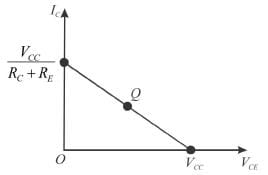# Bipolar Junction Transistor MSQ

## 10 Questions MCQ Test Solid State Physics, Devices & Electronics | Bipolar Junction Transistor MSQ

Description
Attempt Bipolar Junction Transistor MSQ | 10 questions in 45 minutes | Mock test for IIT JAM preparation | Free important questions MCQ to study Solid State Physics, Devices & Electronics for IIT JAM Exam | Download free PDF with solutions
*Multiple options can be correct
QUESTION: 1

### Consider the following statements about an oscillator and choose which of them are true. An oscillator

Solution:

An oscillator is a system consisting of active and passive circuit elements to produce a sinusoidal or other respective waveforms at the output without the amplification of an external source (signal). It transforms the dc power from the source to the ac power in the load.
This consists of a positive feedback amplifier and has noise as the initial signal and consists of a frequency signal selector.
The correct answers are: consists of a positive feedback amplifier, has noise as the initial signal, consists of a frequency selecting network

*Multiple options can be correct
QUESTION: 2

### Which of the following are essential for maintaining oscillations in an oscillator ?

Solution:

For maintaining oscillation in an oscillator, all the four options above are required which are positive feedback, phase shifting network, non-linear biasing circuit and high gain amplifier. Without any one of the above, we will not obtain a sustained oscillation in an oscillator.
The correct answers are: Positive feedback, Design of load (phase shifting network), Non-linear biasing circuit, High gain amplifier

*Multiple options can be correct
QUESTION: 3

### Which of the following are associated with diffusion current.

Solution:

Emitter-base junction current and base-collector current are mainly due to diffusion current.
The correct answers are: emitter-base junction current, base-collector junction current

QUESTION: 4

With the positive probe on an NPN base, an ohmmeter reading between the other transistor terminals should be:

Solution:
*Multiple options can be correct
QUESTION: 5

Choose the following options in respect of a BJT connected in the common-emitter configuration.

Solution:

If BJT connected in common emitter configurations, the power gain is highest, voltage gain is medium, input impedence is low.
The correct answers are: Voltage gain is medium, Low input impedance , Highest power gain

*Multiple options can be correct
QUESTION: 6

The dc load line of an amplifier circuit.

Solution:The dc load line has a negative slope and Q-point lies on this line.
The correct answers are: contains the Q - point, has a negative slope

*Multiple options can be correct
QUESTION: 7

Which of the following factors affect the frequency stability of an oscillator ?

Solution:

The stability of an oscillator depends on (i) inter element capacitances and stray capacitances (ii) temperature variation (iii) size of coil used It does not depend on the load.
The correct answers are: coil size, temperature variation, Inter element capacitances and stray capacitances

*Multiple options can be correct
QUESTION: 8

Which of the following are true in case of negative feedback amplifiers.

Solution:

Negative feedback amplifiers are specifically designed to improve the stability of the amplifier, decrease the distortion of the amplifiers, noise in the amplifier and bandwidth of the amplifier.
The correct answers are: Distortion of the amplifier decreases, Noise of the amplifier decreases, Bandwidth of the amplifier decreases

*Multiple options can be correct
QUESTION: 9

An electronic oscillator gives sustained oscillations when the feedback is.

Solution:

An electronic oscillator gives sustained oscillations when the feedback is negative and in phase with the input.
The correct answers are: negative, in phase with the input

*Multiple options can be correct
QUESTION: 10

If a sinusoidal voltage is applied to the base of a biased npn transistor and resulting sinusoidal collector voltage is clipped near zero volts, the transistor is.

Solution:

Here, the transistor is being driven into saturation which is also a non-linear response. The transistor will not be driven into the cut-off region.
The correct answers are: being driven into stauration, operating non-linearlyUse Code STAYHOME200 and get INR 200 additional OFF Use Coupon Code